``````library(corrplot)
library(psych)
library(ggplot2)
require(gridExtra)
library(car)
library(mice)
library(VIM)
library(caret)
library(dplyr)
library(MASS)``````

# Data Exploration

Here, we read the dataset and shorten the feature names for better readibility in visualizations.

``````df <- read.csv("https://raw.githubusercontent.com/mkivenson/Business-Analytics-Data-Mining/master/Moneyball%20Regression/moneyball-training-data.csv")[-1]
names(df) <- sub("TEAM_", "", names(df))
names(df) <- sub("BATTING_", "bt_", names(df))
names(df) <- sub("BASERUN_", "br_", names(df))
names(df) <- sub("FIELDING_", "fd_", names(df))
names(df) <- sub("PITCHING_", "ph_", names(df))
names(df) <- sub("TARGET_", "", names(df))
``````##   WINS bt_H bt_2B bt_3B bt_HR bt_BB bt_SO br_SB br_CS bt_HBP ph_H ph_HR
## 1   39 1445   194    39    13   143   842    NA    NA     NA 9364    84
## 2   70 1339   219    22   190   685  1075    37    28     NA 1347   191
## 3   86 1377   232    35   137   602   917    46    27     NA 1377   137
## 4   70 1387   209    38    96   451   922    43    30     NA 1396    97
## 5   82 1297   186    27   102   472   920    49    39     NA 1297   102
## 6   75 1279   200    36    92   443   973   107    59     NA 1279    92
##   ph_BB ph_SO fd_E fd_DP
## 1   927  5456 1011    NA
## 2   689  1082  193   155
## 3   602   917  175   153
## 4   454   928  164   156
## 5   472   920  138   168
## 6   443   973  123   149``````

#### Summary

First, we take a look at a summary of the data. A few things of interest are revealed:

• bt_SO, br_SB, br_CS, bt_HBP, ph_SO, and fd_DP have missing values
• The max values of ph_H, ph_BB, ph_SO, and fd_E seem abnormally high
``summary(df)``
``````##       WINS             bt_H          bt_2B           bt_3B
##  Min.   :  0.00   Min.   : 891   Min.   : 69.0   Min.   :  0.00
##  1st Qu.: 71.00   1st Qu.:1383   1st Qu.:208.0   1st Qu.: 34.00
##  Median : 82.00   Median :1454   Median :238.0   Median : 47.00
##  Mean   : 80.79   Mean   :1469   Mean   :241.2   Mean   : 55.25
##  3rd Qu.: 92.00   3rd Qu.:1537   3rd Qu.:273.0   3rd Qu.: 72.00
##  Max.   :146.00   Max.   :2554   Max.   :458.0   Max.   :223.00
##
##      bt_HR            bt_BB           bt_SO            br_SB
##  Min.   :  0.00   Min.   :  0.0   Min.   :   0.0   Min.   :  0.0
##  1st Qu.: 42.00   1st Qu.:451.0   1st Qu.: 548.0   1st Qu.: 66.0
##  Median :102.00   Median :512.0   Median : 750.0   Median :101.0
##  Mean   : 99.61   Mean   :501.6   Mean   : 735.6   Mean   :124.8
##  3rd Qu.:147.00   3rd Qu.:580.0   3rd Qu.: 930.0   3rd Qu.:156.0
##  Max.   :264.00   Max.   :878.0   Max.   :1399.0   Max.   :697.0
##                                   NA's   :102      NA's   :131
##      br_CS           bt_HBP           ph_H           ph_HR
##  Min.   :  0.0   Min.   :29.00   Min.   : 1137   Min.   :  0.0
##  1st Qu.: 38.0   1st Qu.:50.50   1st Qu.: 1419   1st Qu.: 50.0
##  Median : 49.0   Median :58.00   Median : 1518   Median :107.0
##  Mean   : 52.8   Mean   :59.36   Mean   : 1779   Mean   :105.7
##  3rd Qu.: 62.0   3rd Qu.:67.00   3rd Qu.: 1682   3rd Qu.:150.0
##  Max.   :201.0   Max.   :95.00   Max.   :30132   Max.   :343.0
##  NA's   :772     NA's   :2085
##      ph_BB            ph_SO              fd_E            fd_DP
##  Min.   :   0.0   Min.   :    0.0   Min.   :  65.0   Min.   : 52.0
##  1st Qu.: 476.0   1st Qu.:  615.0   1st Qu.: 127.0   1st Qu.:131.0
##  Median : 536.5   Median :  813.5   Median : 159.0   Median :149.0
##  Mean   : 553.0   Mean   :  817.7   Mean   : 246.5   Mean   :146.4
##  3rd Qu.: 611.0   3rd Qu.:  968.0   3rd Qu.: 249.2   3rd Qu.:164.0
##  Max.   :3645.0   Max.   :19278.0   Max.   :1898.0   Max.   :228.0
##                   NA's   :102                        NA's   :286``````

#### Histogram

Next, we create histograms of each of the features and target variable.

• bt_H, bt_2B, bt_BB, br_CS, bt_HBP, fd_DP, WINS all have normal distributions
• ph_H, ph_BB, ph_SO, and fd_E are highly right-skewed
``````grid.arrange(ggplot(df, aes(bt_H)) + geom_histogram(binwidth = 30),
ggplot(df, aes(bt_2B)) + geom_histogram(binwidth = 10),
ggplot(df, aes(bt_3B)) + geom_histogram(binwidth = 10),
ggplot(df, aes(bt_HR)) + geom_histogram(binwidth = 10),
ggplot(df, aes(bt_BB)) + geom_histogram(binwidth = 30),
ggplot(df, aes(bt_SO)) + geom_histogram(binwidth = 50),
ggplot(df, aes(br_SB)) + geom_histogram(binwidth = 30),
ggplot(df, aes(br_CS)) + geom_histogram(binwidth = 10),
ggplot(df, aes(bt_HBP)) + geom_histogram(binwidth = 3),
ggplot(df, aes(ph_H)) + geom_histogram(binwidth = 100),
ggplot(df, aes(ph_HR)) + geom_histogram(binwidth = 10),
ggplot(df, aes(ph_BB)) + geom_histogram(binwidth = 100),
ggplot(df, aes(ph_SO)) + geom_histogram(binwidth = 30),
ggplot(df, aes(fd_E)) + geom_histogram(binwidth = 30),
ggplot(df, aes(fd_DP)) + geom_histogram(binwidth = 10),
ggplot(df, aes(WINS)) + geom_histogram(binwidth = 5),
ncol=4)``````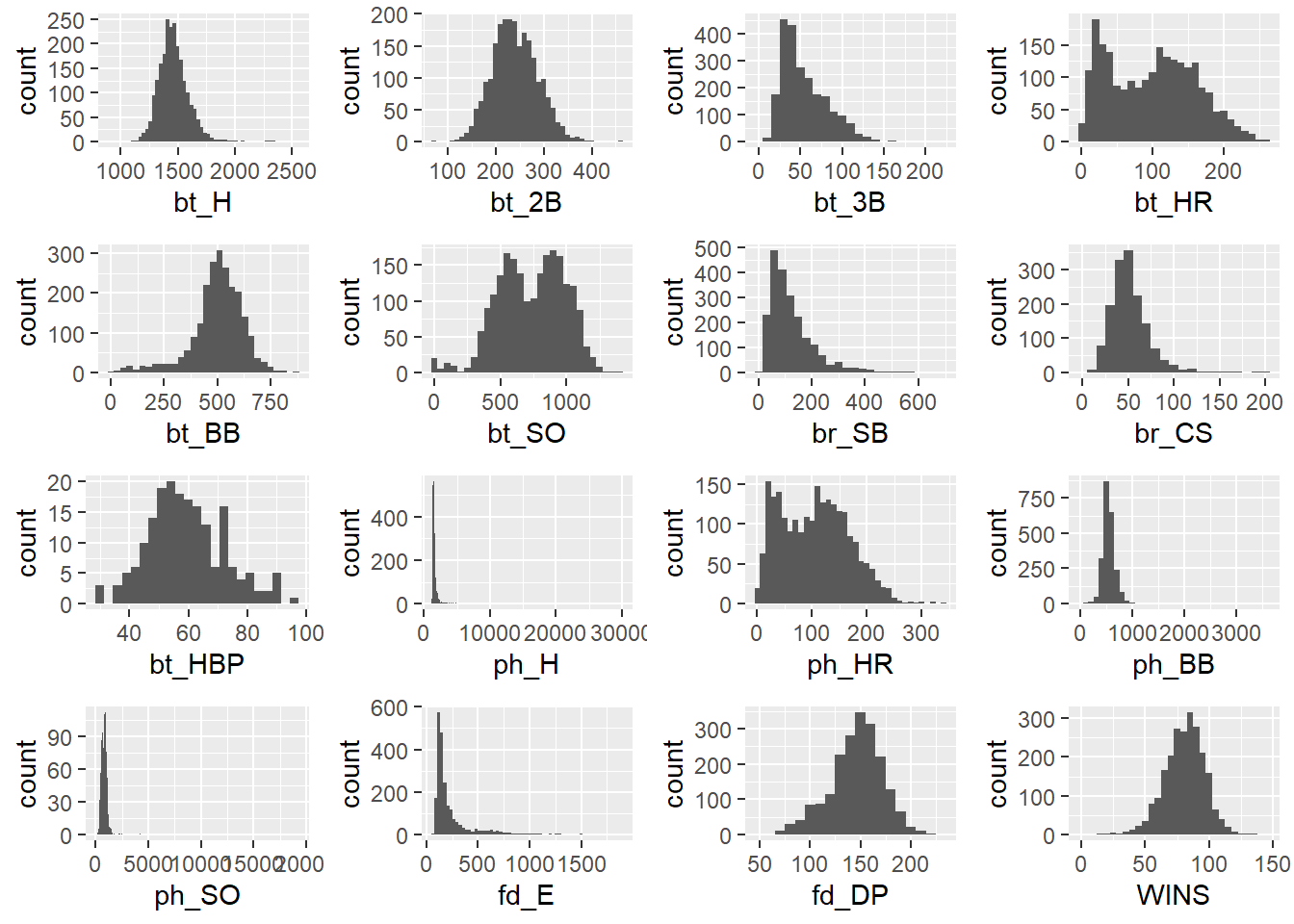#### QQ Plots

• Most of the features are not lined up with the theoretical QQ plot, however this will be addressed by the models we build.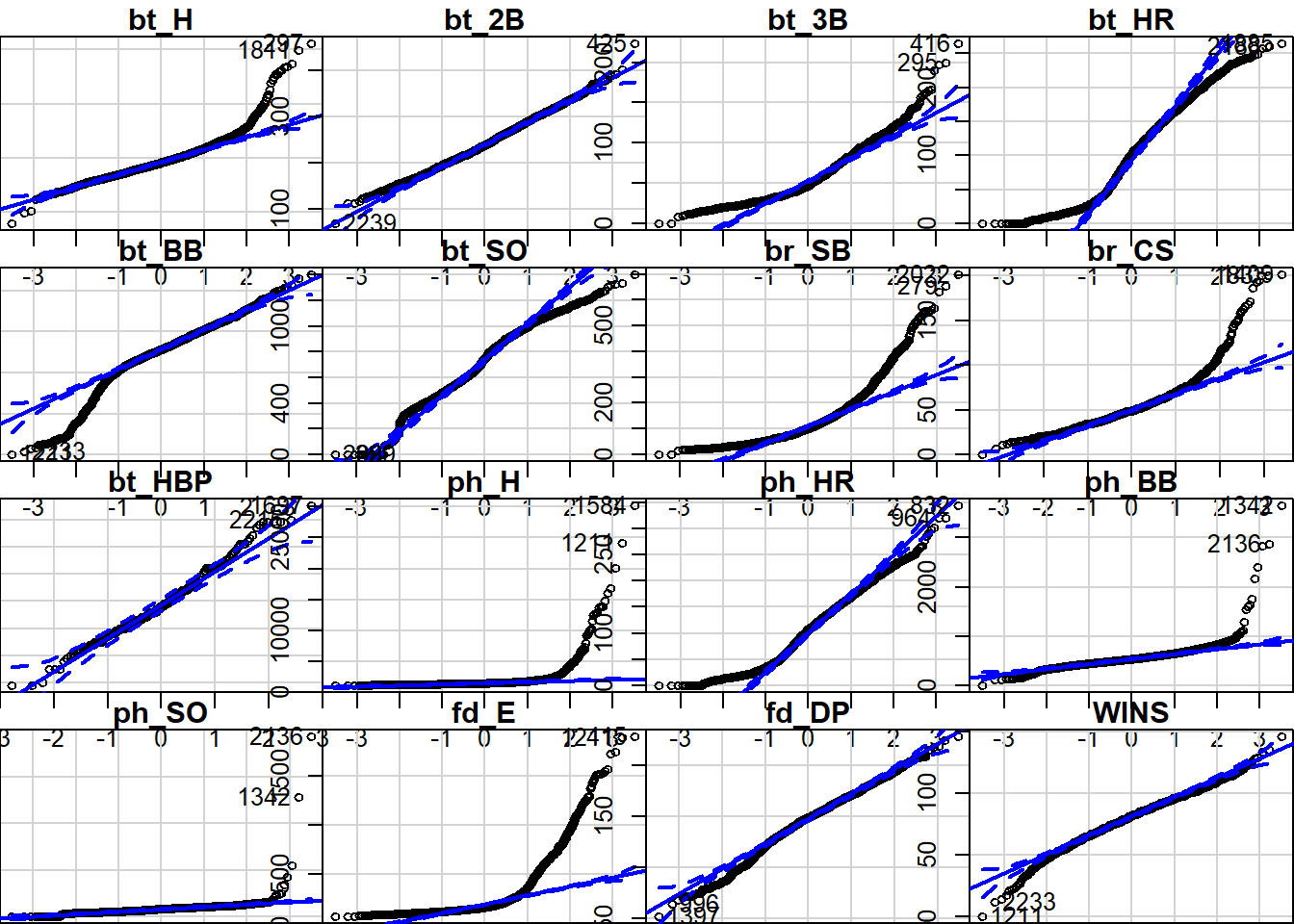#### Boxplot

• Most of the boxplots shown below reflect a long right tail with many outliers.
``````grid.arrange(ggplot(df, aes(x = "bt_H", y = bt_H))+geom_boxplot(),
ggplot(df, aes(x = "bt_2B", y = bt_2B))+geom_boxplot(),
ggplot(df, aes(x = "bt_3B", y = bt_3B))+geom_boxplot(),
ggplot(df, aes(x = "bt_HR", y = bt_HR))+geom_boxplot(),
ggplot(df, aes(x = "bt_BB", y = bt_BB))+geom_boxplot(),
ggplot(df, aes(x = "bt_SO", y = bt_SO))+geom_boxplot(),
ggplot(df, aes(x = "br_SB", y = br_SB))+geom_boxplot(),
ggplot(df, aes(x = "br_CS", y = br_CS))+geom_boxplot(),
ggplot(df, aes(x = "bt_HBP", y = bt_HBP))+geom_boxplot(),
ggplot(df, aes(x = "ph_H", y = ph_H))+geom_boxplot(),
ggplot(df, aes(x = "ph_HR", y = ph_HR))+geom_boxplot(),
ggplot(df, aes(x = "ph_BB", y = ph_BB))+geom_boxplot(),
ggplot(df, aes(x = "ph_SO", y = ph_SO))+geom_boxplot(),
ggplot(df, aes(x = "fd_E", y = fd_E))+geom_boxplot(),
ggplot(df, aes(x = "fd_DP", y = fd_DP))+geom_boxplot(),
ggplot(df, aes(x = "WINS", y = WINS))+geom_boxplot(),
ncol=4)``````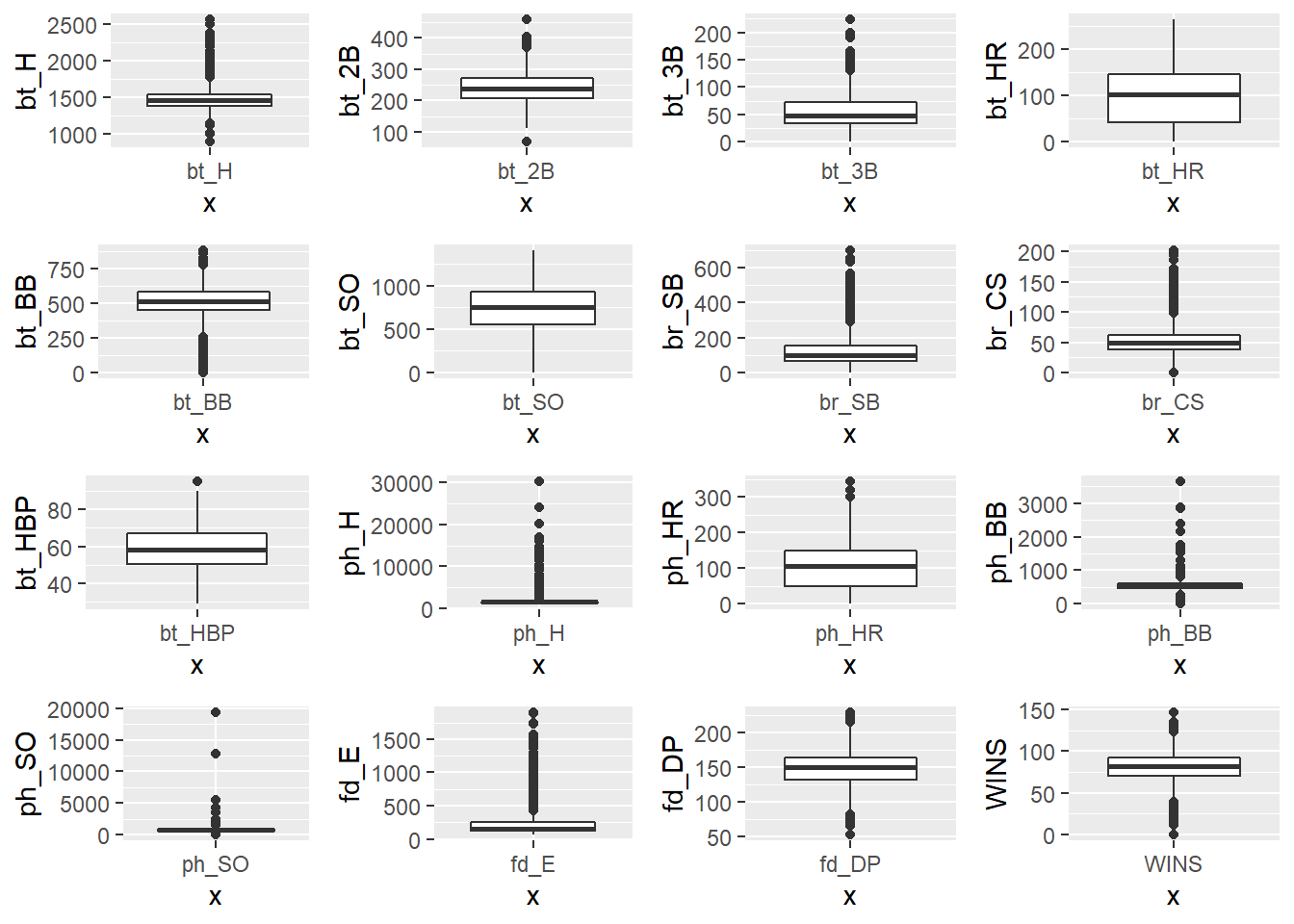#### Correlation Plot

• There is a strong positive correlation between ph_H and bt_H
• There is a strong positive correlation between ph_HR and bt_HR
• There is a strong positive correlation between ph_BB and bt_BB
• There is a strong positive correlation between ph_SO and bt_SO
• There seems to be a weak correlation between bt_HBP/br_SB and Wins
``corrplot(cor(df, use = "complete.obs"), method="color", type="lower", tl.col = "black", tl.srt = 25)``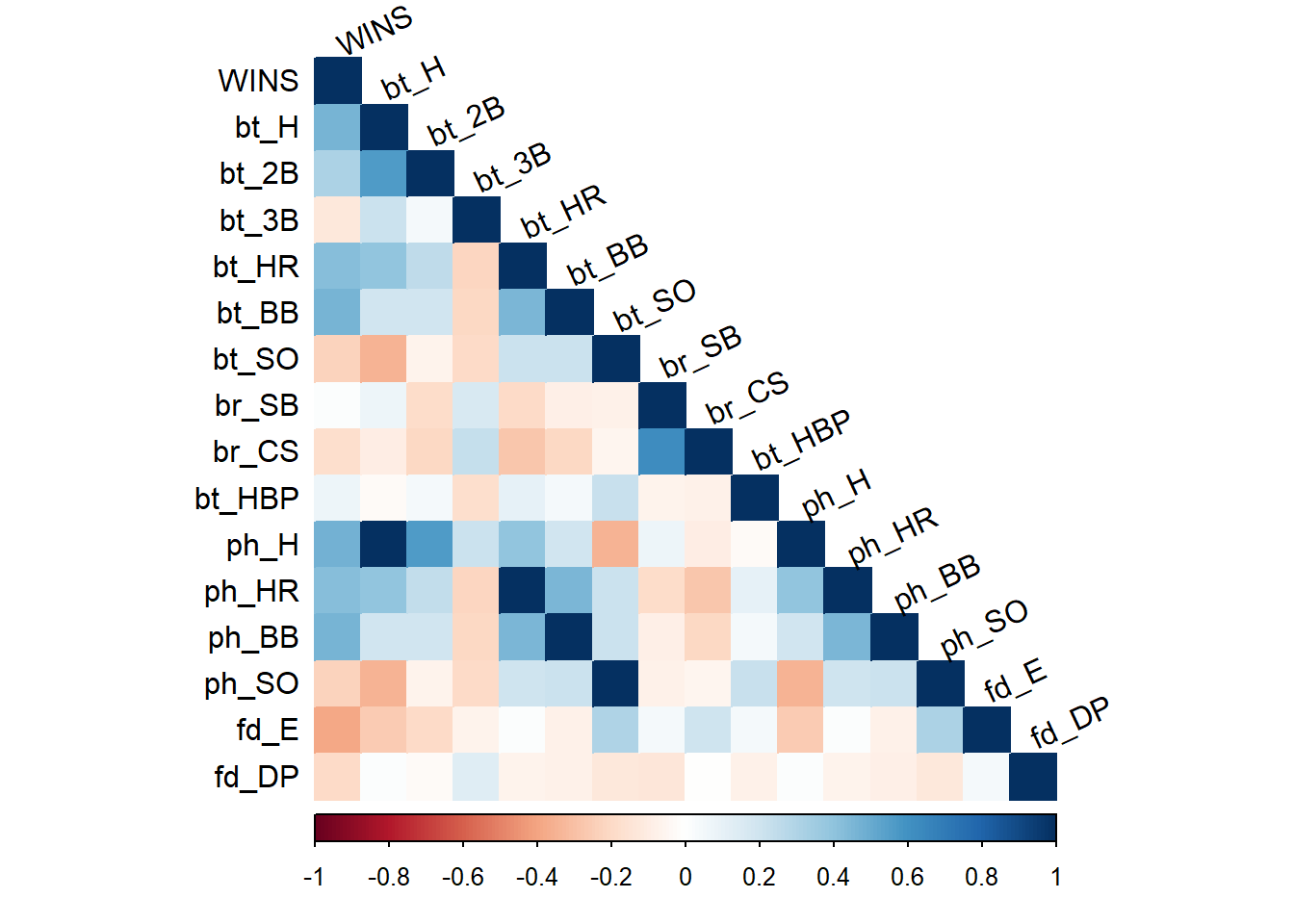#### Scatter Plots

Here, we see a scatter plot of each of the feature variables with the target variable.

``````grid.arrange(ggplot(df, aes(bt_H, WINS)) + geom_point(),
ggplot(df, aes(bt_2B, WINS)) + geom_point(),
ggplot(df, aes(bt_3B, WINS)) + geom_point(),
ggplot(df, aes(bt_HR, WINS)) + geom_point(),
ggplot(df, aes(bt_BB, WINS)) + geom_point(),
ggplot(df, aes(bt_SO, WINS)) + geom_point(),
ggplot(df, aes(br_SB, WINS)) + geom_point(),
ggplot(df, aes(br_CS, WINS)) + geom_point(),
ggplot(df, aes(bt_HBP, WINS)) + geom_point(),
ggplot(df, aes(ph_H, WINS)) + geom_point(),
ggplot(df, aes(ph_HR, WINS)) + geom_point(),
ggplot(df, aes(ph_BB, WINS)) + geom_point(),
ggplot(df, aes(ph_SO, WINS)) + geom_point(),
ggplot(df, aes(fd_E, WINS)) + geom_point(),
ggplot(df, aes(fd_DP, WINS)) + geom_point(),
ncol=4)``````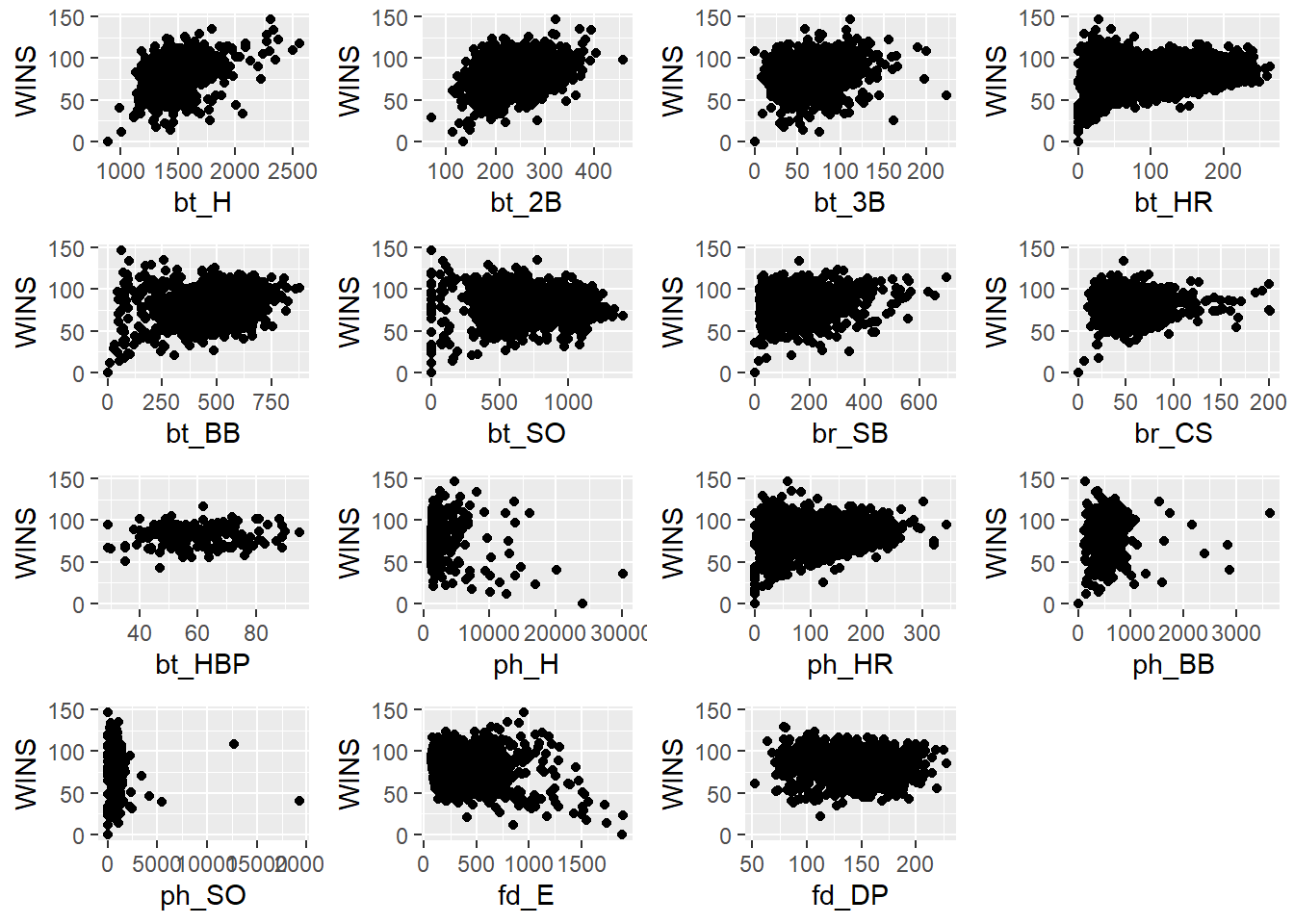# Data Preparation

### Outliers

#### Extreme Values

While exploring the data, we noticed that the max values of ph_H, ph_BB, ph_SO, and fd_E seem abnormally high.

We see that the record for most hits in a season by team (ph_H) was set at 1,724 in 1921. However, we also know that the datapoints were normalized for 162 games in a season. To take a moderate approach, we will remove the some of the most egggregious outliers that are seen in these variables.

``````grid.arrange(ggplot(df, aes(x = "ph_H", y = ph_H))+geom_boxplot(),
ggplot(df, aes(x = "ph_BB", y = ph_BB))+geom_boxplot(),
ggplot(df, aes(x = "ph_SO", y = ph_SO))+geom_boxplot(),
ggplot(df, aes(x = "fd_E", y = fd_E))+geom_boxplot(),
ncol=4)``````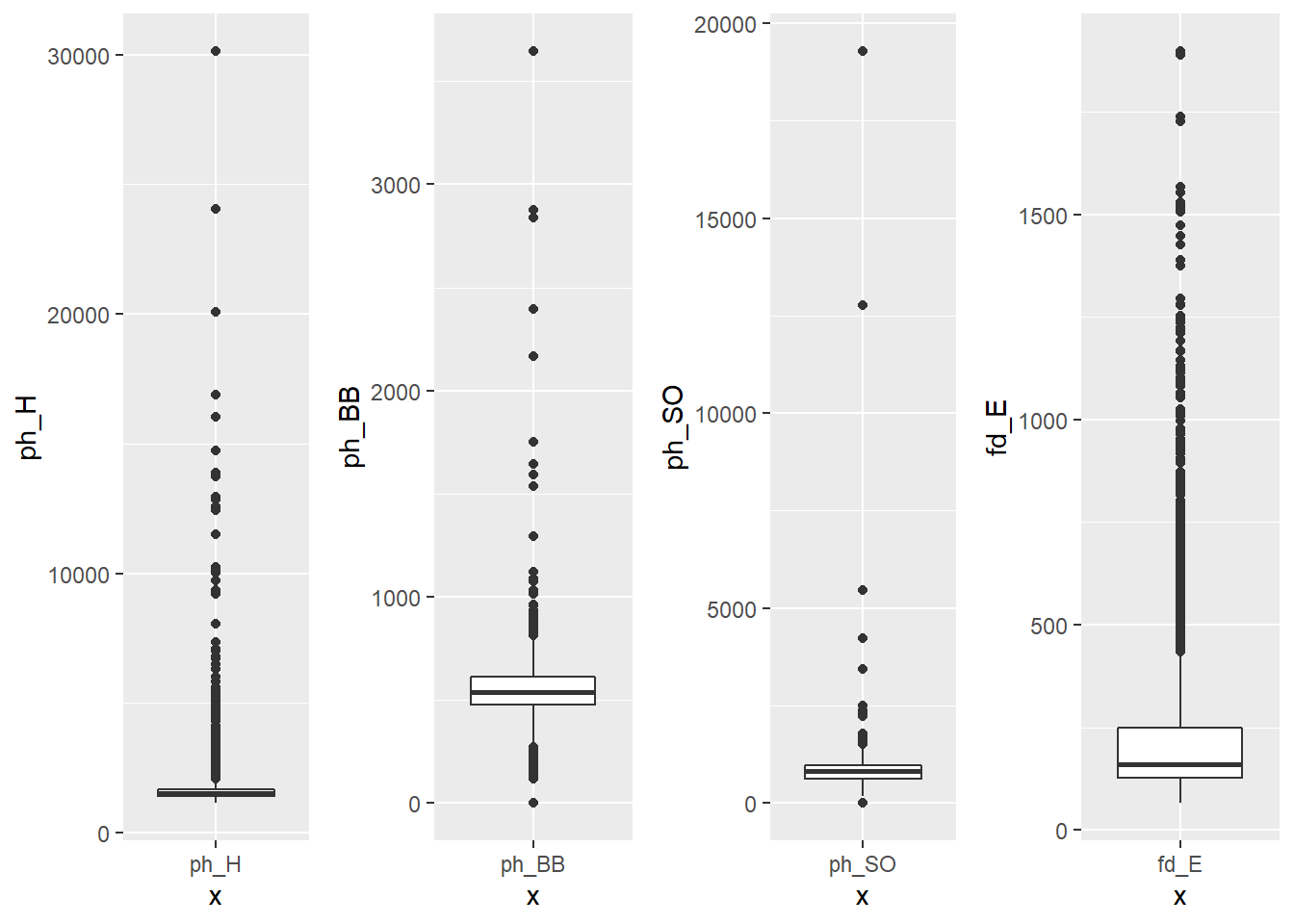``df <- filter(df, ph_H < 15000 | ph_BB < 1500 | ph_SO < 3000 | fd_E < 1500)``

#### Cooks Distance

We will also remove influencial outliers using Cooks distance.

``````mod <- lm(WINS ~ ., data=df)
cooksd <- cooks.distance(mod)
plot(cooksd, pch="*", cex=2, main="Influential Outliers by Cooks distance")
abline(h = 4*mean(cooksd, na.rm=T), col="red")  # add cutoff line
text(x=1:length(cooksd)+1, y=cooksd, labels=ifelse(cooksd>4*mean(cooksd, na.rm=T),names(cooksd),""), col="red")  # add labels``````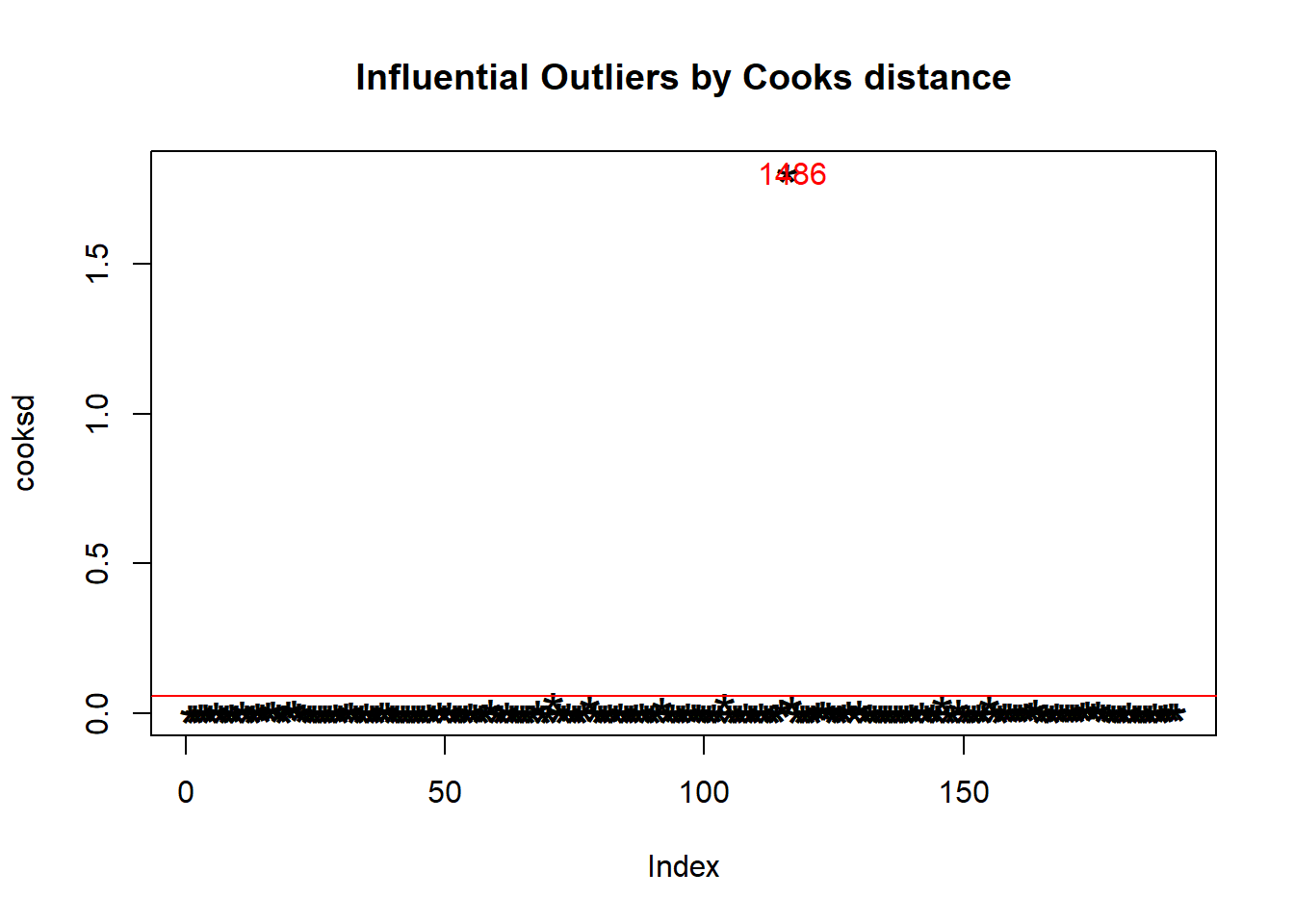We remove the influencial outliers.

``````influential <- as.numeric(names(cooksd)[(cooksd > 4*mean(cooksd, na.rm=T))])
df <- df[-influential, ]``````

### Fill Missing Values

The following features have missing values.

• bt_SO - Strikeouts by batters
• br_SB - Stolen bases
• br_CS - Caught stealing
• bt_HBP - Batters hit by pitch (get a free base)
• ph_SO - Strikeouts by pitchers
• fd_DP - Double Plays

Since most values in bt_HBP are missing (90%), we will drop this feature.

#### Multivariate Imputation by Chained Equations (mice)

We will use Multivariable Imputation by Chained Equations (mice) to fill the missing variables.

``````df <- subset(df, select = -c(bt_HBP))
aggr_plot <- aggr(df, col=c('navyblue','red'), numbers=TRUE, sortVars=TRUE, labels=names(data), cex.axis=.7, gap=3, ylab=c("Histogram of missing data","Pattern"))``````